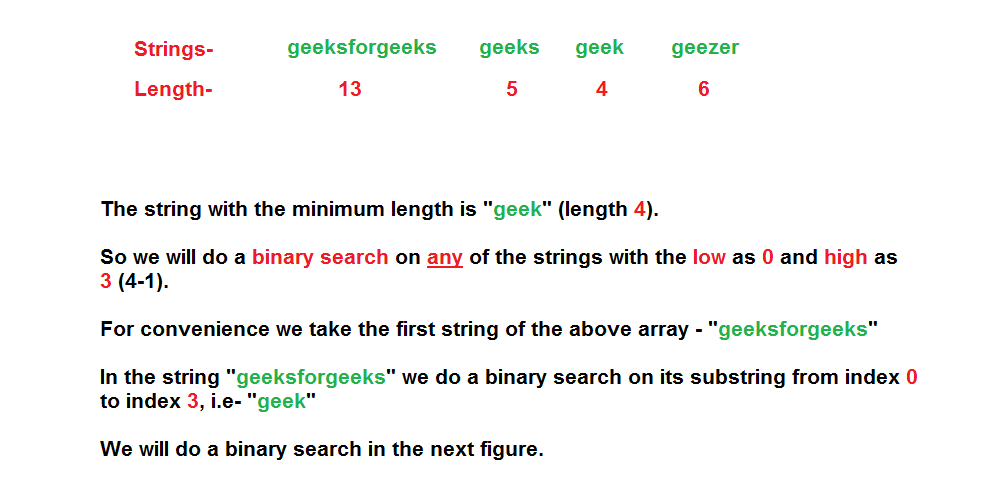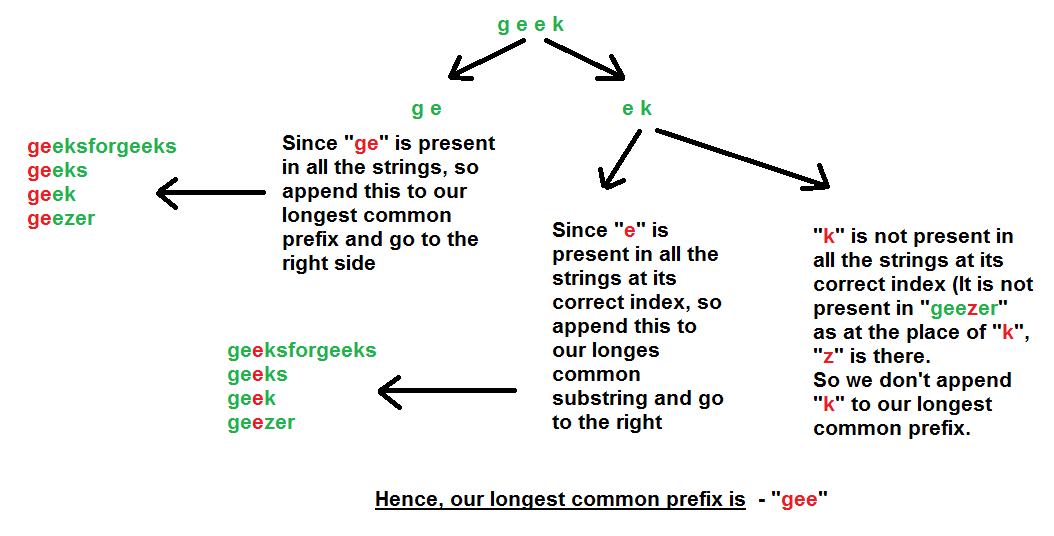# Longest Common Prefix using Binary Search

• Difficulty Level : Medium
• Last Updated : 21 Jan, 2022

Given a set of strings, find the longest common prefix.

```Input  : {“geeksforgeeks”, “geeks”, “geek”, “geezer”}
Output : "gee"

Input  : {"apple", "ape", "april"}
Output : "ap"

Input  : {"abcd"}
Output : "abcd"```

Previous Approaches – Word by Word Matching , Character by Character Matching, Divide and Conquer
Steps:

1. Find the string having the minimum length. Let this length be L.
2. Perform a binary search on any one string (from the input array of strings). Let us take the first string and do a binary search on the characters from the index – 0 to L-1.
3. Initially, take low = 0 and high = L-1 and divide the string into two halves – left (low to mid) and right (mid+1 to high).
4. Check whether all the characters in the left half is present at the corresponding indices (low to mid) of all the strings or not. If it is present then we append this half to our prefix string and we look in the right half in a hope to find a longer prefix.(It is guaranteed that a common prefix string is there.)
5. Otherwise, if all the characters in the left half is not present at the corresponding indices (low to mid) in all the strings, then we need not look at the right half as there is some character(s) in the left half itself which is not a part of the longest prefix string. So we indeed look at the left half in a hope to find a common prefix string. (It may be possible that we don’t find any common prefix string)

Algorithm Illustration considering strings as – “geeksforgeeks”, “geeks”, “geek”, “geezer”Below is the implementation of above approach.

## C++

 `//  A C++ Program to find the longest common prefix``#include``using` `namespace` `std;` `// A Function to find the string having the minimum``// length and returns that length``int` `findMinLength(string arr[], ``int` `n)``{``    ``int` `min = INT_MAX;` `    ``for` `(``int` `i=0; i<=n-1; i++)``        ``if` `(arr[i].length() < min)``            ``min = arr[i].length();``    ``return``(min);``}` `bool` `allContainsPrefix(string arr[], ``int` `n, string str,``                       ``int` `start, ``int` `end)``{``    ``for` `(``int` `i=0; i<=n-1; i++)``        ``for` `(``int` `j=start; j<=end; j++)``            ``if` `(arr[i][j] != str[j])``                ``return` `(``false``);``    ``return` `(``true``);``}` `// A Function that returns the longest common prefix``// from the array of strings``string commonPrefix(string arr[], ``int` `n)``{``    ``int` `index = findMinLength(arr, n);``    ``string prefix; ``// Our resultant string` `    ``// We will do an in-place binary search on the``    ``// first string of the array in the range 0 to``    ``// index``    ``int` `low = 0, high = index;` `    ``while` `(low <= high)``    ``{``        ``// Same as (low + high)/2, but avoids overflow``        ``// for large low and high``        ``int` `mid = low + (high - low) / 2;` `        ``if` `(allContainsPrefix (arr, n, arr, low, mid))``        ``{``            ``// If all the strings in the input array contains``            ``// this prefix then append this substring to``            ``// our answer``            ``prefix = prefix + arr.substr(low, mid-low+1);` `            ``// And then go for the right part``            ``low = mid + 1;``        ``}` `        ``else` `// Go for the left part``            ``high = mid - 1;``    ``}` `    ``return` `(prefix);``}` `// Driver program to test above function``int` `main()``{``    ``string arr[] = {``"geeksforgeeks"``, ``"geeks"``,``                    ``"geek"``, ``"geezer"``};``    ``int` `n = ``sizeof` `(arr) / ``sizeof` `(arr);` `    ``string ans = commonPrefix(arr, n);` `    ``if` `(ans.length())``        ``cout << ``"The longest common prefix is "``             ``<< ans;``    ``else``        ``cout << ``"There is no common prefix"``;``    ``return` `(0);``}`

## Java

 `// A Java Program to find the longest common prefix` `class` `GFG {` `    ``// A Function to find the string having the``    ``// minimum length and returns that length``    ``static` `int` `findMinLength(String arr[], ``int` `n)``    ``{``        ``int` `min = Integer.MAX_VALUE;``        ``for` `(``int` `i = ``0``; i <= (n - ``1``); i++)``        ``{``            ``if` `(arr[i].length() < min) {``                ``min = arr[i].length();``            ``}``        ``}``        ``return` `min;``    ``}` `    ``static` `boolean` `allContainsPrefix(String arr[], ``int` `n,``                         ``String str, ``int` `start, ``int` `end)``    ``{``        ``for` `(``int` `i = ``0``; i <= (n - ``1``); i++)``        ``{``            ``String arr_i = arr[i];``            ` `            ``for` `(``int` `j = start; j <= end; j++)``                ``if` `(arr_i.charAt(j) != str.charAt(j))``                    ``return` `false``;``        ``}``        ``return` `true``;``    ``}` `    ``// A Function that returns the longest common prefix``    ``// from the array of strings``    ``static` `String commonPrefix(String arr[], ``int` `n)``    ``{``        ``int` `index = findMinLength(arr, n);``        ``String prefix = ``""``; ``// Our resultant string` `        ``// We will do an in-place binary search on the``        ``// first string of the array in the range 0 to``        ``// index``        ``int` `low = ``0``, high = index-``1``;``        ``while` `(low <= high) {``            ` `            ``// Same as (low + high)/2, but avoids``            ``// overflow for large low and high``            ``int` `mid = low + (high - low) / ``2``;` `            ``if` `(allContainsPrefix(arr, n, arr[``0``], low,``                                                  ``mid))``            ``{``                ``// If all the strings in the input array``                ``// contains this prefix then append this``                ``// substring to our answer``                ``prefix = prefix + arr[``0``].substring(low,``                                          ``mid + ``1``);` `                ``// And then go for the right part``                ``low = mid + ``1``;``            ``}``            ``else` `// Go for the left part``            ``{``                ``high = mid - ``1``;``            ``}``        ``}` `        ``return` `prefix;``    ``}` `    ``// Driver program to test above function``    ``public` `static` `void` `main(String args[])``    ``{``        ``String arr[] = {``"geeksforgeeks"``, ``"geeks"``,``                               ``"geek"``, ``"geezer"``};``        ``int` `n = arr.length;` `        ``String ans = commonPrefix(arr, n);``        ` `        ``if` `(ans.length() > ``0``)``            ``System.out.println(``"The longest common"``                            ``+ ``" prefix is "` `+ ans);``        ``else``            ``System.out.println(``"There is no common"``                                      ``+ ``" prefix"``);``    ``}``}` `// This code is contributed by Indrajit Sinha.`

## Python3

 `# A Python3 Program to find``# the longest common prefix` `# A Function to find the string having the``# minimum length and returns that length``def` `findMinLength(strList):``    ``return` `len``(``min``(arr, key ``=` `len``))` `def` `allContainsPrefix(strList, ``str``,``                      ``start, end):``    ``for` `i ``in` `range``(``0``, ``len``(strList)):``        ``word ``=` `strList[i]``        ``for` `j ``in` `range``(start, end ``+` `1``):``            ``if` `word[j] !``=` `str``[j]:``                ``return` `False``    ``return` `True` `# A Function that returns the longest``# common prefix from the array of strings``def` `CommonPrefix(strList):``    ``index ``=` `findMinLength(strList)``    ``prefix ``=` `""     ``# Our resultant string` `    ``# We will do an in-place binary search``    ``# on the first string of the array``    ``# in the range 0 to index``    ``low, high ``=` `0``, index ``-` `1``    ``while` `low <``=` `high:` `        ``# Same as (low + high)/2, but avoids``        ``# overflow for large low and high``        ``mid ``=` `int``(low ``+` `(high ``-` `low) ``/` `2``)``        ``if` `allContainsPrefix(strList,  ``                             ``strList[``0``], low, mid):``            ` `            ``# If all the strings in the input array``            ``# contains this prefix then append this``            ``# substring to our answer``            ``prefix ``=` `prefix ``+` `strList[``0``][low:mid ``+` `1``]` `            ``# And then go for the right part``            ``low ``=` `mid ``+` `1``        ``else``:``            ` `            ``# Go for the left part``            ``high ``=` `mid ``-` `1` `    ``return` `prefix` `# Driver Code``arr ``=` `[``"geeksforgeeks"``, ``"geeks"``,``       ``"geek"``, ``"geezer"``]``lcp ``=` `CommonPrefix(arr)` `if` `len``(lcp) > ``0``:``    ``print` `(``"The longest common prefix is "` `+``                                 ``str``(lcp))``else``:``    ``print` `(``"There is no common prefix"``)` `# This code is contributed by garychan8523`

## C#

 `// C# Program to find the longest common prefix using System;``using` `System;               ``public` `class` `GFG {`` ` `    ``// A Function to find the string having the``    ``// minimum length and returns that length``    ``static` `int` `findMinLength(``string` `[]arr, ``int` `n)``    ``{``        ``int` `min = ``int``.MaxValue;``        ``for` `(``int` `i = 0; i <= (n - 1); i++)``        ``{``            ``if` `(arr[i].Length < min) {``                ``min = arr[i].Length;``            ``}``        ``}``        ``return` `min;``    ``}`` ` `    ``static` `bool` `allContainsPrefix(``string` `[]arr, ``int` `n,``                         ``string` `str, ``int` `start, ``int` `end)``    ``{``        ``for` `(``int` `i = 0; i <= (n - 1); i++)``        ``{``            ``string` `arr_i = arr[i];``             ` `            ``for` `(``int` `j = start; j <= end; j++)``                ``if` `(arr_i[j] != str[j])``                    ``return` `false``;``        ``}``        ``return` `true``;``    ``}`` ` `    ``// A Function that returns the longest common prefix``    ``// from the array of strings``    ``static` `string` `commonPrefix(``string` `[]arr, ``int` `n)``    ``{``        ``int` `index = findMinLength(arr, n);``        ``string` `prefix = ``""``; ``// Our resultant string`` ` `        ``// We will do an in-place binary search on the``        ``// first string of the array in the range 0 to``        ``// index``        ``int` `low = 0, high = index;``        ``while` `(low <= high) {``             ` `            ``// Same as (low + high)/2, but avoids``            ``// overflow for large low and high``            ``int` `mid = low + (high - low) / 2;`` ` `            ``if` `(allContainsPrefix(arr, n, arr, low,``                                                  ``mid))``            ``{``                ``// If all the strings in the input array``                ``// contains this prefix then append this``                ``// substring to our answer``                ``prefix = prefix + arr.Substring(low,``                                          ``mid + 1);`` ` `                ``// And then go for the right part``                ``low = mid + 1;``            ``}``            ``else` `// Go for the left part``            ``{``                ``high = mid - 1;``            ``}``        ``}`` ` `        ``return` `prefix;``    ``}`` ` `    ``// Driver program to test above function``    ``public` `static` `void` `Main()``    ``{``        ``string` `[]arr = {``"geeksforgeeks"``, ``"geeks"``,``                               ``"geek"``, ``"geezer"``};``        ``int` `n = arr.Length;` `        ``string` `ans = commonPrefix(arr, n);``         ` `        ``if` `(ans.Length > 0)``            ``Console.WriteLine(``"The longest common"``                            ``+ ``" prefix is - "` `+ ans);``        ``else``            ``Console.WriteLine(``"There is no common"``                                      ``+ ``" prefix"``);``    ``}``}`` ` `// This code is contributed by PrinciRaj1992`

## Javascript

 ``

Output :

`The longest common prefix is - gee`

Time Complexity :
The recurrence relation is

`T(M) = T(M/2) + O(MN) `

where

```N = Number of strings
M = Length of the largest string```

So we can say that the time complexity is O(NM log M)
Auxiliary Space: To store the longest prefix string we are allocating space which is O(N) where, N = length of the largest string among all the strings
This article is contributed by Rachit Belwariar. If you like GeeksforGeeks and would like to contribute, you can also write an article using write.geeksforgeeks.org or mail your article to review-team@geeksforgeeks.org. See your article appearing on the GeeksforGeeks main page and help other Geeks.